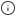## Introduction

Estimate the value at a given percentile, or the percentile rank of a given value, using the t-digest algorithm. This estimation is more memory- and CPU-efficient than an exact calculation using PostgreSQL's `percentile_cont` and `percentile_disc` functions.

`tdigest` is one of two advanced percentile approximation aggregates provided in TimescaleDB Toolkit. It is a space-efficient aggregation, and it provides more accurate estimates at extreme quantiles than traditional methods.

`tdigest` is somewhat dependent on input order. If `tdigest` is run on the same data arranged in different order, the results should be nearly equal, but they are unlikely to be exact.

The other advanced percentile approximation aggregate is `uddsketch`, which produces stable estimates within a guaranteed relative error. If you aren't sure which to use, try the default percentile estimation method, `percentile_agg`. It uses the `uddsketch` algorithm with some sensible defaults.## Functions in this group

### Aggregate

tdigest
Aggregate data in a `tdigest` for further calculation of percentile estimates

### Accessor

approx_percentile
Estimate the value at a given percentile from a `tdigest`
approx_percentile_rank
Estimate the percentile of a given value from a `tdigest`
mean
Calculate the exact mean from values in a `tdigest`
num_vals
Get the number of values contained in a `tdigest`

### Rollup

rollup
Roll up multiple `tdigest`s

## Function details

`tdigest(  buckets INTEGER,  value DOUBLE PRECISION) RETURNS TDigest`

This is the first step for calculating approximate percentiles with the `tdigest` algorithm. Use `tdigest` to create an intermediate aggregate from your raw data. This intermediate form can then be used by one or more accessors in this group to compute final results.

Optionally, multiple such intermediate aggregate objects can be combined using `rollup()` before an accessor is applied.

Required arguments
NameTypeDescription
`buckets``INTEGER`Number of buckets in the digest. Increasing this provides more accurate quantile estimates, but requires more memory.
`value``DOUBLE PRECISION`Column of values to aggregate for the `tdigest` object.
Returns
ColumnTypeDescription
`tdigest``TDigest`A percentile estimator object created to calculate percentiles using the `tdigest` algorithm
Examples

Given a table called `samples`, with a column called `data`, build a `tdigest` using the `data` column. Use 100 buckets for the approximation:

`SELECT tdigest(100, data) FROM samples;`
`approx_percentile(  percentile DOUBLE PRECISION,  tdigest  TDigest) RETURNS DOUBLE PRECISION`

Estimate the approximate value at a percentile from a `tdigest` aggregate.

Required arguments
NameTypeDescription
`percentile``DOUBLE PRECISION`The percentile to compute. Must be within the range `[0.0, 1.0]`.
`tdigest``TDigest`The `tdigest` aggregate.
Returns
ColumnTypeDescription
`approx_percentile``DOUBLE PRECISION`The estimated value at the requested percentile.
Examples

Estimate the value at the first percentile, given a sample containing the numbers from 0 to 100:

`SELECT  approx_percentile(0.01, tdigest(data))FROM generate_series(0, 100) data;`
`approx_percentile-------------------            0.999`
`approx_percentile_rank(  value DOUBLE PRECISION,  digest TDigest) RETURNS DOUBLE PRECISION`

Estimate the the percentile at which a given value would be located.

Required arguments
NameTypeDescription
`value``DOUBLE PRECISION`The value to estimate the percentile of.
`digest``TDigest`The `tdigest` aggregate.
Returns
ColumnTypeDescription
`approx_percentile_rank``DOUBLE PRECISION`The estimated percentile associated with the provided value.
Examples

Estimate the percentile rank of the value `99`, given a sample containing the numbers from 0 to 100:

`SELECT  approx_percentile_rank(99, tdigest(data))FROM generate_series(0, 100) data;`
`approx_percentile_rank----------------------------        0.9851485148514851`
`mean(  digest TDigest) RETURNS DOUBLE PRECISION`

Calculate the exact mean of the values in a `tdigest` aggregate. Unlike percentile calculations, the mean calculation is exact. This accessor allows you to calculate the mean alongside percentiles, without needing to create two separate aggregates from the same raw data.

Required arguments
NameTypeDescription
`digest``TDigest`The `tdigest` aggregate to extract the mean from.
Returns
ColumnTypeDescription
`mean``DOUBLE PRECISION`The mean of the values in the `tdigest` aggregate.
Examples

Calculate the mean of the integers from 0 to 100:

`SELECT mean(tdigest(data))FROM generate_series(0, 100) data;`
`mean------50`
`num_vals(  digest TDigest) RETURNS DOUBLE PRECISION`

Get the number of values contained in a `tdigest` aggregate. This accessor allows you to calculate a count alongside percentiles, without needing to create two separate aggregates from the same raw data.

Required arguments
NameTypeDescription
`digest``TDigest`The `tdigest` aggregate to extract the number of values from.
Returns
ColumnTypeDescription
`num_vals``DOUBLE PRECISION`The number of values in the `tdigest` aggregate.
Examples

Count the number of integers from 0 to 100:

`SELECT num_vals(tdigest(data))FROM generate_series(0, 100) data;`
`num_vals-----------    101`
`rollup(  digest TDigest) RETURNS TDigest`

Combine multiple intermediate `tdigest` aggregates, produced by `tdigest`, into a single intermediate `tdigest` aggregate. For example, you can use `rollup` to combine `tdigest`s from 15-minute buckets into daily buckets.

Required arguments
NameTypeDescription
`digest``TDigest`The `tdigest`s to roll up.
Returns
ColumnTypeDescription
`rollup``TDigest`A new `tdigest` created by combining the input `tdigests`.

## Extended examples

### Aggregate and roll up percentile data to calculate daily percentiles

Create an hourly continuous aggregate that contains a percentile aggregate:

`CREATE MATERIALIZED VIEW foo_hourlyWITH (timescaledb.continuous)AS SELECT    time_bucket('1 h'::interval, ts) as bucket,    tdigest(value) as tdigestFROM fooGROUP BY 1;`

You can use accessors to query directly from the continuous aggregate for hourly data. You can also roll the hourly data up into daily buckets, then calculate approximate percentiles:

`SELECT    time_bucket('1 day'::interval, bucket) as bucket,    approx_percentile(0.95, rollup(tdigest)) as p95,    approx_percentile(0.99, rollup(tdigest)) as p99FROM foo_hourlyGROUP BY 1;`

Keywords